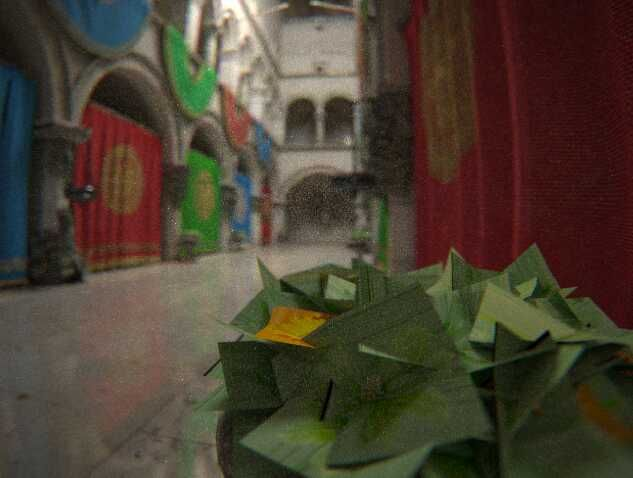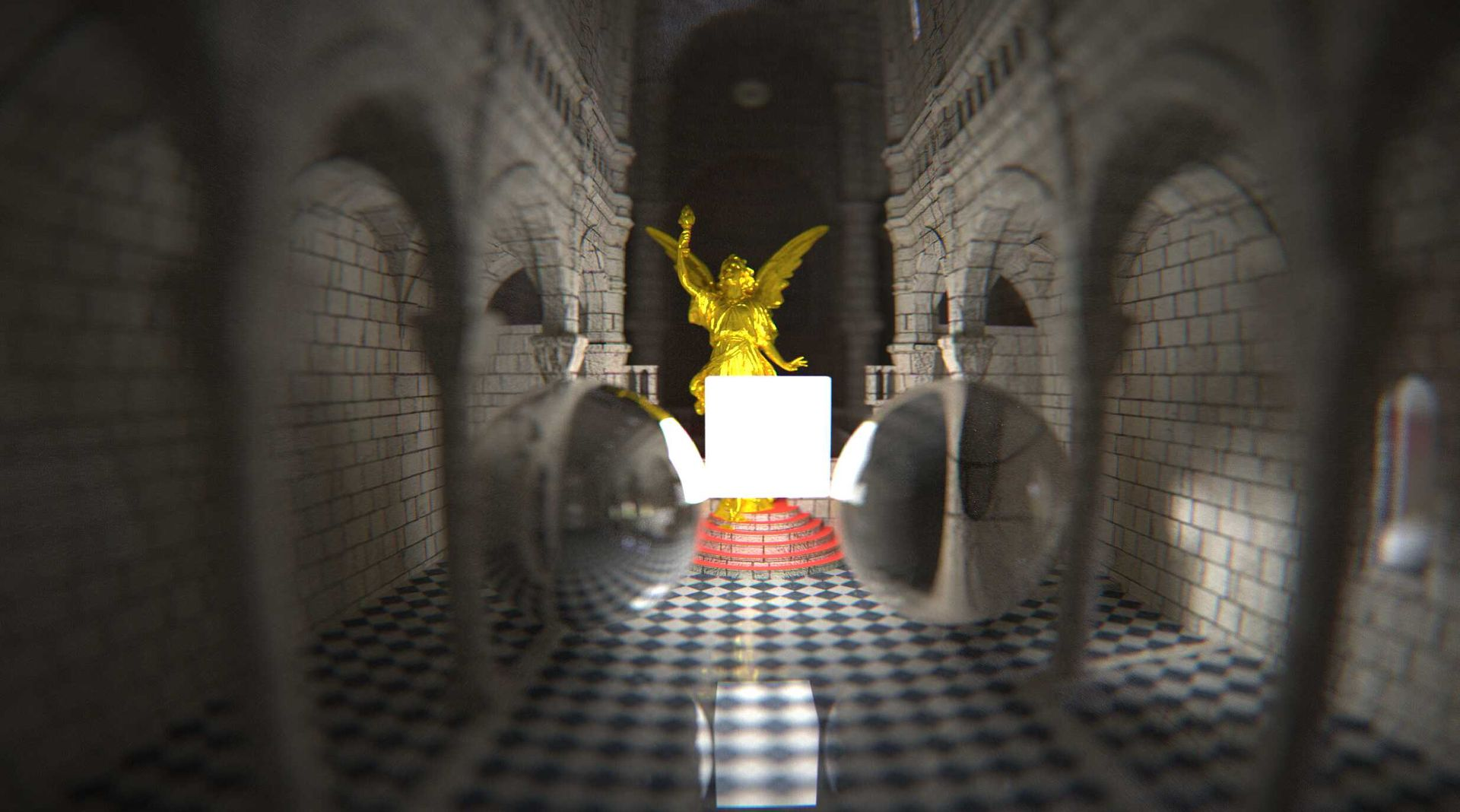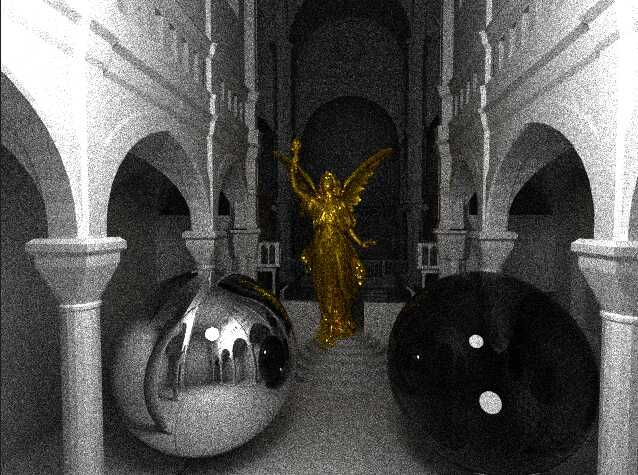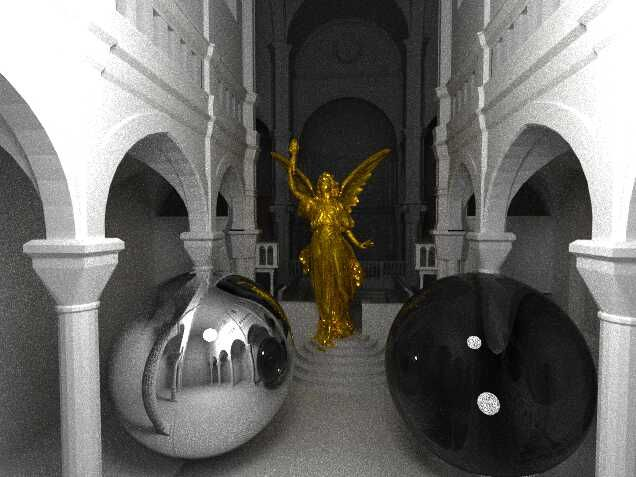C++
cuda

# Shining light on a die

Today we are going to dive into the wonderous world of pathtraced rendering. This technique has long since transcended animated movies and is becoming more and more a viable option for real time applications.

In short, pathtracing attempts to first formulate a complex integral that describes how much light arrives at any point in the scene and then solve it stochastically using Monte Carlo. The rendering equation is a complex beast to read...

$$L_{\text{o}}(\mathbf {x} ,\omega _{\text{o}},\lambda ,t)=L_{\text{e}}(\mathbf {x} ,\omega _{\text{o}},\lambda ,t)\ +\int _{\Omega }f_{\text{r}}(\mathbf {x} ,\omega _{\text{i}},\omega _{\text{o}},\lambda ,t)L_{\text{i}}(\mathbf {x} ,\omega _{\text{i}},\lambda ,t)(\omega _{\text{i}}\cdot \mathbf {n} )\operatorname {d} \omega _{\text{i}}}$$ ..but it looks more daunting than it is.

$$L_{\text{o}}$$ describes amount of energy that a point $$o$$ emmits (called irradiance).

$$L_\text{e}$$ is simply the amount of light produced by o directly (making it a light source).

Then we see an integral over the hemisphere above that point, containing another set of functions. This integral describes all the light that this point reflects.

$$f_{\text{r}}$$ is a function that describes the relation between the incoming and outgoing light. Properties like the color of the material and how much light it absorbs are represented by this function.

$$L_{\text{i}}$$ is a recursive call to the rendering equation. In other words, we also evaluate how much light arrives a point i before being able to calculate how much light it reflects to o.

Finally $$\omega _{\text{i}} \cdot {\mathbf n}$$ is just the cosine between the surface normal and the incoming light, correcting for the fact that light that arrives under an angle is spread out over a larger surface and thus contributes less to the theorical singularity o for which we want to calculate the incoming energy. Formally this term converts the radiance to irradiance.

If you are a pure maths fan who solves integrals analytically for breakfast, then you probably felt your cortisol level rise when I mentioned a recursive integral. Luckily, if you are willing to get your hands dirty, then it turns out that solving it numerically is not actually that hard. We also will not be exploring the whole process of pathtracing in detail, but focus especially on a little trick to help converge our stochastic experiment a bit faster, which I don't see mentioned often.

But before we go that route, I think I owe you a window into to future that justifies why we are going through all this. What we want to be able to do is realistically render how light interacts with a scene and get images like the following.The source code for the program (written in C++ and cuda) can be found over on my github.

## Solving integrals with Monte Carlo sampling

Hopefully these images have excited you enough to work through a bit more maths. Namely, how in the hell we are going to solve the rendering equation?

The first insight we need is that we can take random samples uniformly over the domain of the integral. After enough samples, the average will converge to the true solution of the integral.

Secondly, for the nested integral we can simply continue taking another random sample over its domain. Even though it is a nested integral, the expected value of the chained experiment is still correct.

Lastly, we are not required to sample the domain of the integral uniformly, as long as we correct for the fact that we have 'bias' to certain subsections of the domain. In fact, we can use any Probability Density Function (PDF) we like, as long as it never zero (unless the integral itself is also zero there) and integrates to one (we want to be energy preserving after all).

The person who taught me this (Jacco Bikker) has explained it beautifully on his own blog if that went a little too fast.

## NEE? Yes!

Next Event Estimation (NEE) is a wonderful little trick which bring us very close to the trick I'd like to show you today. The point of NEE is to sample direct and indirect light separately. As most of the energy will come from direct light it makes sense to sample with a higher probability making our pathtracer converge faster. To do this we effectively split the PDF in two, and cheat a little bit by assuming that the irradiance of surface from which we sample our direct light is dominated by its own emission term. (i.e. a light bulb doesn't appear brighter when you turn on another light). This allows us to get much less variance with few samples:Sampling direct light can be done the other way around; instead of sampling a random direction on the hemisphere we can randomly choose a point on an emissive triangle and trace it back to our point o to ensure that it's not occluded by other objects in the scene.

## Don't you turn your back on me!

The cost of pathtracing used to originate mostly from the scene intersection procedure that helps us find point i after selecting a random direction to sample the hemisphere (or light source directly). Lately, with dedicated hardware support like Nvidia's RTX cores it's actually the cost of shading that has taken over. Whatever way you put it though, it's clear that shading and intersecting together make up most of the costs.

Consequently, that gives us quite a reasonable budget when generating samples to do some extra work if it decreases variance. And we still have an efficiency problem when sampling direct light. Namely, light sources themselves can be meshes (like the cube in the in the first set of images) and as is usually the case with meshes, we have a notion of the outside and inside, determined by the winding order of vertices. As most meshes are closed this means that we will never ever receive light from the backface of a triangle (the light doesn't escape the closed mesh). Therefore, after we have chosen a light vertex to sample direct light, we can first determine if we are perhaps looking at the backface of this triangle, in which case we can just report 0 energy received without doing any expensive occlusion tests!

## The trick, Monte Carlo inside Monte Carlo

But we can do more! Since it is relatively cheap to inspect said facts of a random emissive triangle, we can do another little Monte Carlo experiment. Instead of picking just one light, we pick say four (with replacement), and remember for how many of these four we are looking at there front face. We then use the first of these 4 that had a successful front face test (if any, otherwise contribution is still 0) to actually do the occlusion test and calculate the true contribution. This drastically improves the chance of finding a possible light source that can contribute light, but by itself introduces bias. After all, we simply picked the first light for which the front face test passed. However, we also know an estimate for the prior probability that this could have happened (1/4, 2/4 or 3/4). So we can correct for this bias by multiplying with this prior probability. Over time, the average of this rough estimate will also converge to its true value, ensuring that our pathtracer still converges to the analytical solution of the rendering equation!

I thought that was pretty neat :)